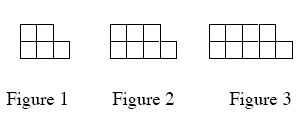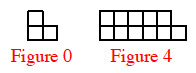### Home > AC > Chapter 4 > Lesson 4.1.3 > Problem4-21

4-21.

Two of the connections in your representations web are pattern → table and pattern → rule. Practice these connections as you answer the questions below.1. On graph paper, draw Figure 0 and Figure 4 for the pattern at below.

What is the growth between the number of tiles in each figure?2. Represent the number of tiles in each figure with a table.

$\left. \begin{array} { | c | c | c | c | c | c | } \hline \text { Figure } & { 0 } & { 1 } & { 2 } & { 3 } & { 4 } \\ \hline \text { Tile } & { 3 } & { 5 } & { 7 } & { 9 } & { 11 } \\ \hline \end{array} \right.$

3. Represent the number of tiles in each figure with an algebraic rule.

What is the growth between the number of tiles in each figure?

What is the starting amount?

$y=2x+3$